Courses

# Evaluation of Integrals - Mathematical Methods of Physics, UGC - NET Physics Physics Notes | EduRev

## Physics : Evaluation of Integrals - Mathematical Methods of Physics, UGC - NET Physics Physics Notes | EduRev

The document Evaluation of Integrals - Mathematical Methods of Physics, UGC - NET Physics Physics Notes | EduRev is a part of the Physics Course Physics for IIT JAM, UGC - NET, CSIR NET.
All you need of Physics at this link: Physics

Complex Integrals

We now turn our attention to the problem of integrating complex functions.  We will find that integrals of analytic functions are well behaved and that many properties from calculus carry over to the complex case.

We introduce the integral of a complex function by defining the integral of a complex-valued function of a real variable

Definition

6.1 (Definite Integral of a Complex Integrand).  Let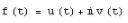where u(t) and v(t) are real-valued functions of the real variable t for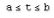.  Then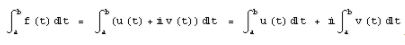.        (6-1)

We generally evaluate integrals of this type by finding the antiderivatives of u(t) and v(t) and evaluating the definite integrals on the right side of Equation (6-1).  That is, if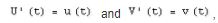,  we have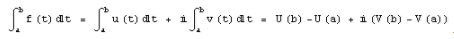.        (6-2)

Example 6.1.  Show that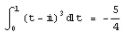.

Solution.  We write the integrand in terms of its real and imaginary parts, i.e.,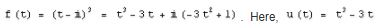and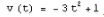.  The integrals of u(t) and v(t) are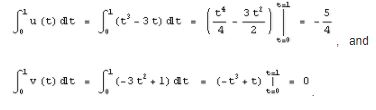.

Hence, by Definition (6-1),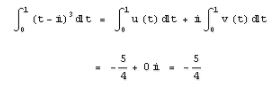Example 6.2.  Show that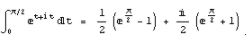.

Solution :  We use the method suggested by Definitions (6-1) and (6-2).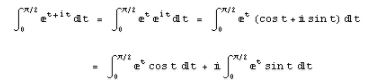We can evaluate each of the integrals via integration by parts.  For example,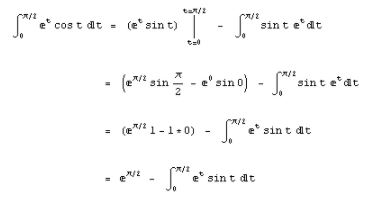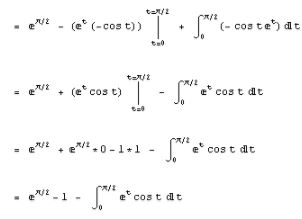Adding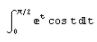to both sides of this equation and then dividing by 2 gives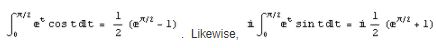.  Therefore,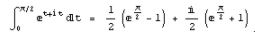.

Complex integrals have properties that are similar to those of real integrals.  We now trace through several commonalities.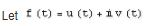and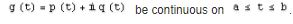.

Using Definition (6-1), we can easily show that the integral of their sum is the sum of their integrals, that is

.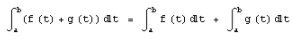(6-3)

If we divide the interval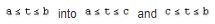and integrate f(t) over these subintervals by using (6-1), then we get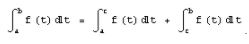.                           (6-4)

Similarly, if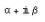denotes a complex constant, then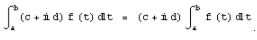.                  (6-5)

If the limits of integration are reversed, then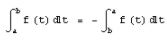.                          (6-6)

The integral of the product f(t)g(t) becomes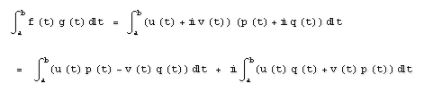(6-7)

Example 6.3.  Let us verify property (6-5).  We start by writing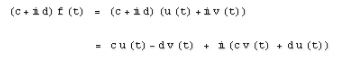Using Definition (6-1), we write the left side of Equation (6-5) as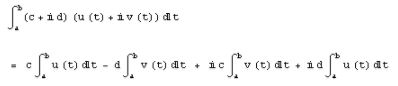which is equivalent to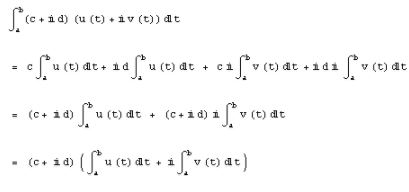Therefore,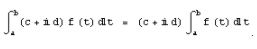.

It is worthwhile to point out the similarity between equation (6-2) and its counterpart in calculus.  Suppose that U and V are differentiable on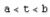and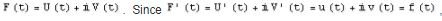,  equation (6-2) takes on the familiar form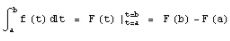.               (6-8)

where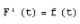.  We can view Equation (6-8) as an extension of the fundamental theorem of calculus.  In Section 6.4 we show how to generalize this extension to analytic functions of a complex variable.  For now, we simply note an important case of Equation (6-8):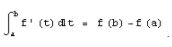.                 (6-9)

Example 6.4.  Use Equation (6-8) to show that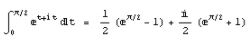.

Solution.  We seek a function F with the property that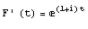.  We note that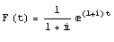satisfies this requirement, so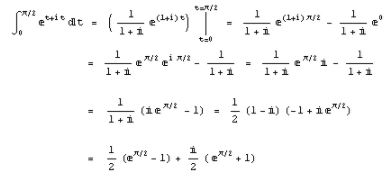which is the same result we obtained in Example 6.2, but with a lot less work.

Remark 6.1  Example 6.4 illustrates the potential computational advantage we have when we lift our sights to the complex domain.  Using ordinary calculus techniques to evaluate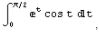, for example, required a lengthy integration by parts procedure (Example 6.2).  When we recognize this expression as the real part of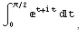, however, the solution comes quickly.  This is just one of the many reasons why good physicists and engineers, in addition to mathematicians, benefit from a thorough working knowledge of complex analysis.

Offer running on EduRev: Apply code STAYHOME200 to get INR 200 off on our premium plan EduRev Infinity!

159 docs

,

,

,

,

,

,

,

,

,

,

,

,

,

,

,

,

,

,

,

,

,

,

,

,

;